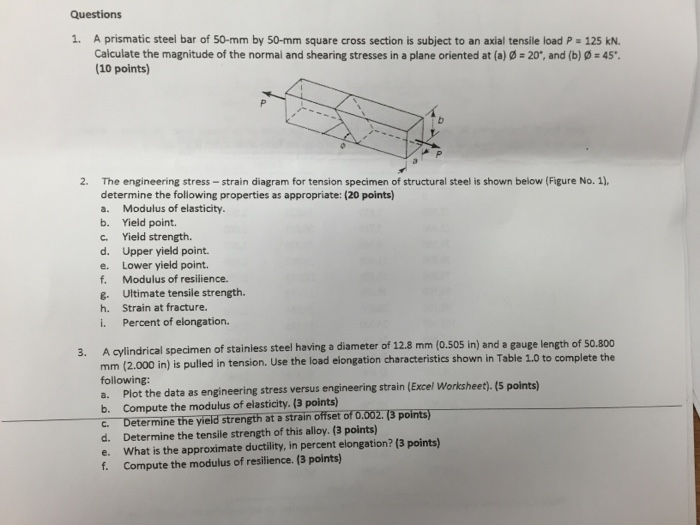### Create an Account

Already have account?

### Forgot Your Password ?

Home / Questions / Image for Questions 1 A prismatic steel bar of 50 mm by 50 mm square cross section is subj...

# Image for Questions 1 A prismatic steel bar of 50 mm by 50 mm square cross section is subject to an axial tensile load Show transcribed image text Questions 1 A prismatic steel bar of 50 mm byShow transcribed image text Questions 1. A prismatic steel bar of 50-mm by 50-mm square cross section is subject to an axial tensile load P = 125 kN. Calculate the magnitude of the normal and shearing stresses in a plane oriented at (a) phi = 20 degree, and (b) phi = 45 degree. 2 The engineering stress - strain diagram for tension specimen of structural steel is shown below, determine the following properties at appropriate: a. Modulus of elasticity. b. Yield point. C. Yield strength. d. Upper yield point. e. Lower yield point. f. Modulus of resilience. g. Ultimate tensile strength. h. Strain at fracture. i. Percent of elongation. 3. A cylindrical specimen of stainless steel having a diameter of 12.8 mm (0.505 in) and a gauge length of 50.800 mm (2.000 in) is pulled in tension. Use the load elongation characteristics shown in Table 1.0 to complete the following: a. Plot the data as engineering stress versus engineering strain (Excel Worksheet). b. Compute the modulus of elasticity. c. Determine the yield strength at a strain offset of 0.002. d. Determine the tensile strength of this alloy. e. What is the approximate ductility, in percent elongation? f. Compute the modulus of resilience.

Jun 19 2020 View more View Less

#### Answer (Solved)Subscribe To Get Solution